# Funderstanding competitive neural networks

Funderstanding is a little term I came up with a few years ago for fun ways of understanding complex concepts. The typical university way of teaching something is by laying the theoretical groundwork (hours of boredom), rehashing the elementary subjects you need to know (hours of more boredom) and then eventually providing an explanation for what’s going on (another few hours of boredom), after which you leave with no more understanding than you had in the beginning. Opposed to this, when you’re trying to funderstand something, you start with a fun motivational example before drilling down to the why and how of it all.

This is the first post in a series of three, and intended to be the ‘fun introduction’ to a particularly interesting topic: competitive neural networks and their use in vector quantisation (please, please stop running, I know that sounds like heavy maths but I promise I’ll keep that to a minimum!). In Part 2, we’ll discuss something called Self-Organising Feature Maps (SOFMs), and thereafter, we’ll look at Growing Neural Gas in Part 3.

### Vector quantization: the general idea

Imagine you have a black-and-white image. You can think of such an image as, effectively, a list of point coordinates (x, y) for every point you want to be coloured black. You would then approach a grid, like in a square-ruled mathematics exercise book, and colour in every point on the list. It stands to reason that the more points you have, the longer your list would have to be. For this example, I have digitised my work badge headshot, and created just such a list for it, with a relatively low density – it gave me about 15,000 (!) points: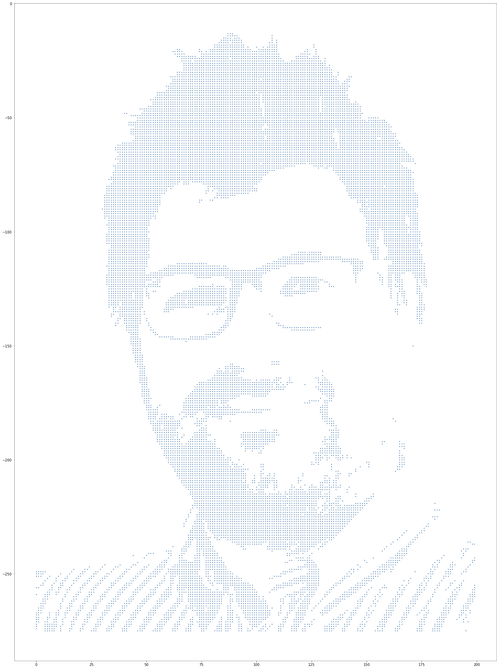My work badge photo, digitized, converted to black and white and thresholded for optimum performance.

The question is, can we reduce the number of points and still get a recognisable, semantically faithful image? One solution would be to find points that represent a bunch of points in their vicinity fairly well, and use them as a ‘proxy’ for those points. Instead of using 15,000 points, we would designate, say, a few hundred new points, and put them in places so that they are each relatively representative of the points surrounding them. This would then allow us to replace all those points with the nearest new point, effectively reducing 15,000 points to a couple of hundred. What’s more, we can create a list of the new points — this is often called a ‘code book’ — , and instead of having to put down coordinates each time, we would simply replace the (x, y) coordinate pair for each point with the index of the closest code book entry. If we have set our number of points well, then we will get a pretty decent approximation.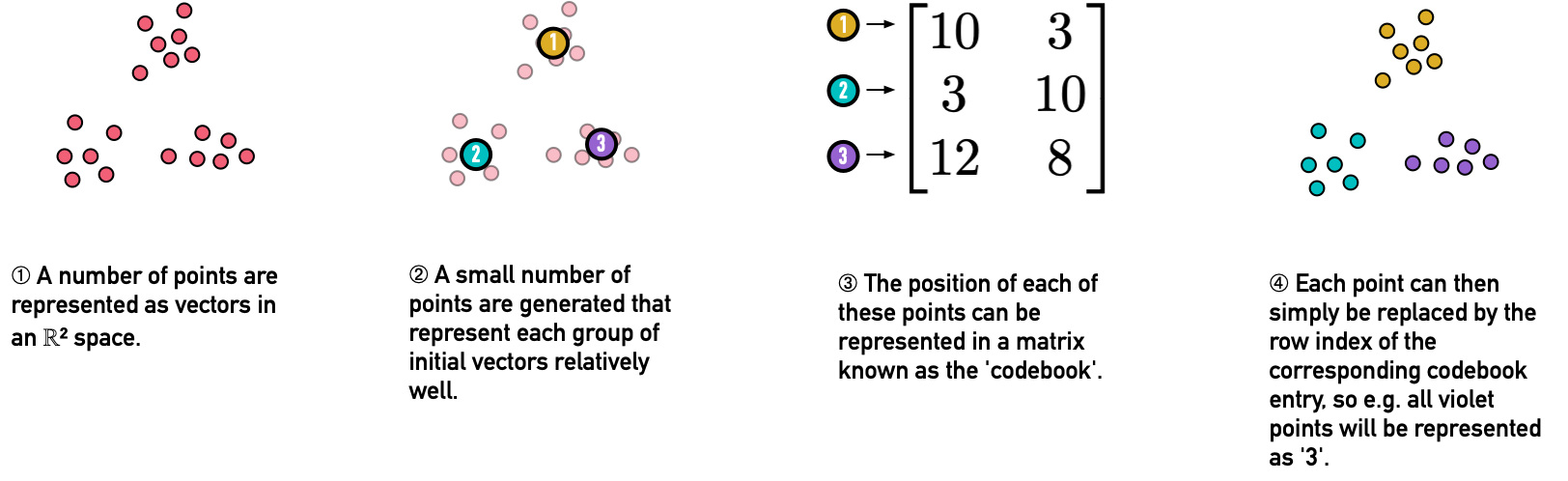The underlying idea of vector quantization: represent a large number of vectors by a smaller number of entries in a codebook, each of which is a relatively good proxy for the points it represents.

There is but one question left: how do we exactly do this? I mean, great idea, but we need to have a way to find those points, right? The easiest way is, of course, to define an error function and keep optimising until we get there.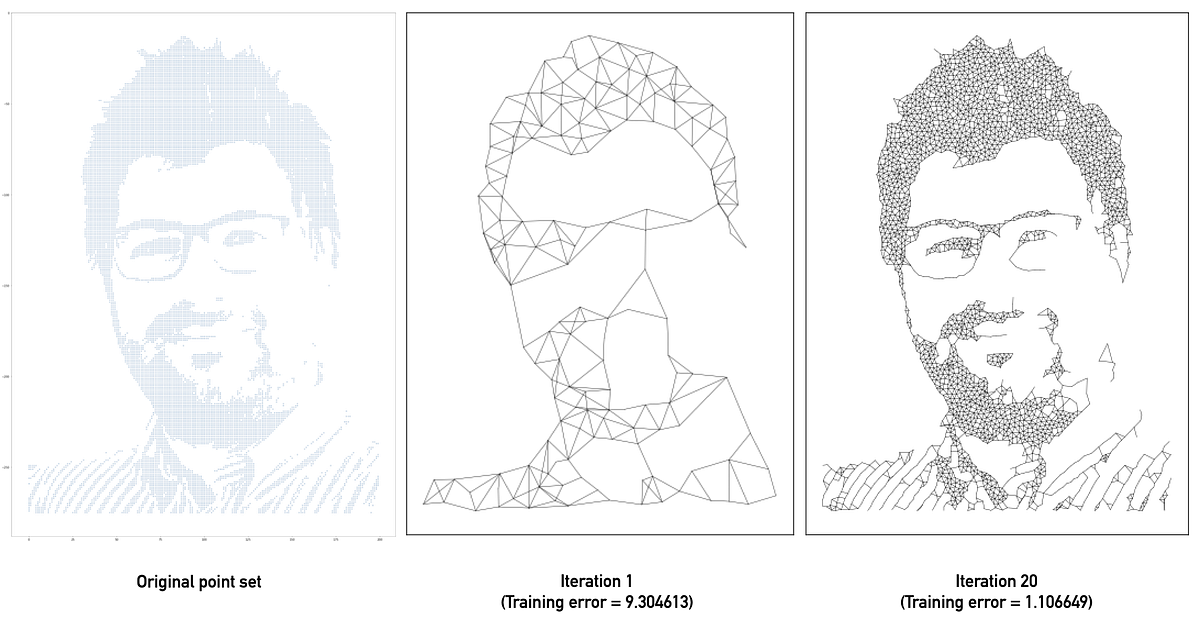The Growing Neural Gas algorithm converges to the point set relatively quickly: in 20 iterations, it created a semantically comprehensible representation, still with only 2,500 points — 16% of the original 15,564 points.

One approach would be to simply drop random points, calculate how many new points they represent and how many of the points in their neighbourhood are already represented versus how many points in their neighbourhood are not supposed to be represented at all. We then keep adding new points and throwing out badly performing ones. This is generally similar to two older methods of machine learning known as Simple Competitive Hebbian Learning. The problem is that these can take ages to converge. And I do mean ages — and most of the time, the results are not all too impressive.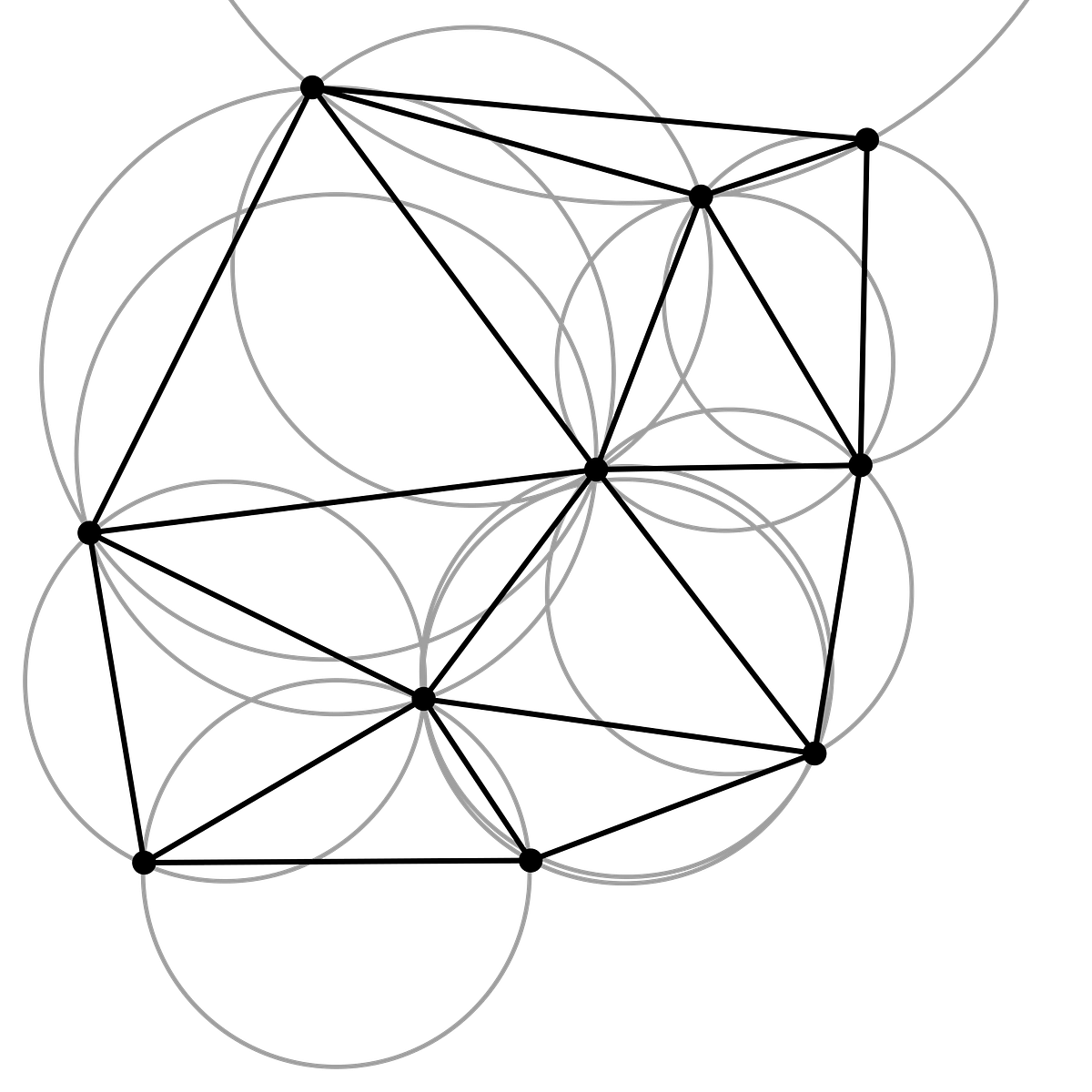The idea of Delaunay triangulation: to divided the set of points into triangles so that no point is within the circumcircle of another triangle (gray). By definition, this also means a Delaunay triangulation does not have intersections between the triangulating lines. Illustration courtesy of Wikipedia/Gjacquenot.

Instead, we can do one better. We have some mathematical tricks up our sleeve that help us with this, called triangulations. Triangulations basically divide a space into triangles in a particular way. With points, the simplest triangulation is of course start connecting points until you get a lot of triangles. Turns out, there are smarter ways to do that. Delaunay triangulation creates triangles between a number of points so that no other point is within the circumcircle (the circle comprising all three points of the triangle) of any triangle. This gives us a non-intersecting triangle mesh. A fantastic little side effect is that if we connect the centres of the circumcircles, we get what is called the Voronoi partitioning of the space circumscribed by the points. The borders of every Voronoi partition will be drawn so that the Voronoi partition around the point P will comprise every point that is closer to P than to any other point in the initial point set. That helps us divide space fairly well between points: we can measure the effectiveness of our model by simply measuring what percentage of points are within the Voronoi partitions inside the code book points’ grid and what percentage of it is empty. This makes for a relatively easy-to-optimise error function. One good thing is that both Delaunay triangulation and Voronoi partitioning generalise really well into higher dimensions, so whatever works in two-dimensional space can also be used in higher dimensional spaces.

### Getting competitive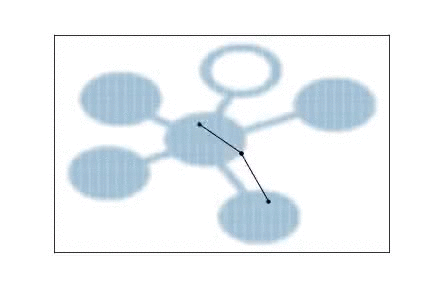A Growing Neural Gas model learning the topology of the Starschema logo, 100 iterations with a high drop-out rate.

Okay, so what about ‘competitive’ neural networks? Most neural networks you may have encountered follow a certain pattern: neurons with activation functions get inputs and produce outputs. In that sense, ‘competitive’ neural networks are quite different. Rather, in a competitive neural network, the neurons ‘compete’ to be activated, where activation is usually a function of distance from a selected data point. The neuron closest to the data point — that is, with the highest activation — ‘wins’, and is moved towards the data point, attracting some of its neighbourhood. In this sense, competitiveness allows learning topology, a useful side effect when used to reconstruct higher-dimensional shapes from a lower-dimensional representation. Indeed, Martinetz and Schulten, who created the first simple Neural Gas model, called it a topology-representing network (TRN). This paper, by Marco Piastra, shows the impressive potential of competitive neural networks to recreate even very complex shapes, such as a 22-genus heptoroid or the Stanford bunny.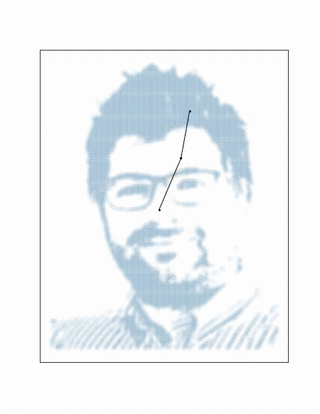Neural gas models can learn fairly complex topologies, such as the human face, as seen before. In this example, a high-dropout slow-converging Growing Neural Gas starting with two vertices and adding a new one every ten iterations creates an approximation of my photo. This graph contains only 752 nodes, a compression of 95.17% of the original 15,564 points.

Competitive learning has a fairly close neurophysiologial analogy. It is a form of Hebbian learning. This approach to machine learning derives from the observation that ‘neurons that fire together wire together’: that is, when independent neurons respond to stimuli simultaneously, synaptic strength (a measure of neuronal ‘closeness’) is increased. It is this feature of neurons that is leveraged by Growing Neural Gas to connect individual vertices and spares us having to specify a map size and shape as Self-Organizing Feature Maps do: best matches and second best matches are connected as ‘co-responders’ and the strength of the connection depends on the relative strength of response between the two points. If both points respond strongly, it indicates they were almost equidistant to the data point, and therefore can be treated as closely connected — ‘firing together’ causes the algorithms to ‘wire together’ the relevant points.

While it may sound abstract, these algorithms can be easily adapted to play a part in our day-to-day machine learning workload. First and most important among the many applications within unsupervised learning in which Growing Neural Gas and Self-Organising Feature Maps are useful is, of course, clustering. But unlike many clustering algorithms, Growing Neural Gas for instance does not need to be provided the number of clusters in advance. This can be useful where the number of disjoint clusters is the question to begin with. For example, consider the problem of counting the number of words on a page: Growing Neural Gas, if well-configured, can join the words into individual subgraphs, and the number of disconnected subgraphs gives the word count. Similarly, multiple high-dimensional clusters that are hard to visualise and interpret can be easily counted using Growing Neural Gas based algorithms.

The two implementations we will discuss in the following parts of this series — Growing Neural Gas and Self-Organising Feature Maps (Kohonen maps) — have a range of uses. They can be used not only for reproducing shapes but also for clustering and embedding high-dimensional data sets, as we will see. Because these techniques belong to the wider area of topological data analysis, which can get extremely maths-intensive, and because they diverge from the way we commonly understand neural networks as consisting of layers of feed-forward neurons and connections between them trained e.g. using backpropagation. These algorithms have also been unduly neglected despite their fascinating properties since their discovery in the 1990s. With modern tensor algebra tools such as Numpy, Theano and Tensorflow at our disposal, this is the best time to dive into competitive neural networks and realise their immense potential. In the next installation of this series, we will be discussing Self-Organising Feature Maps first, and how they can be used for more day-to-day data science applications.

The next part of this series, Part 2: Self-Organising Feature Maps, will appear on 20 January 2019. Watch this space!